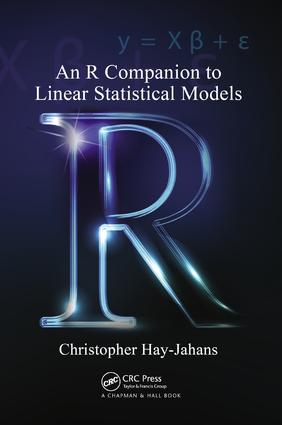An R Companion to Linear Statistical Models

1st Edition

Chapman and Hall/CRC

372 pages | 97 B/W Illus.

Paperback: 9781138116030
pub: 2017-10-18
\$82.95
x
Hardback: 9781439873656
pub: 2011-10-19
Currently out of stock
\$220.00
x
eBook (VitalSource) : 9780429064364
pub: 2011-10-19
from \$110.00

FREE Standard Shipping!

Description

Focusing on user-developed programming, An R Companion to Linear Statistical Models serves two audiences: those who are familiar with the theory and applications of linear statistical models and wish to learn or enhance their skills in R; and those who are enrolled in an R-based course on regression and analysis of variance. For those who have never used R, the book begins with a self-contained introduction to R that lays the foundation for later chapters.

This book includes extensive and carefully explained examples of how to write programs using the R programming language. These examples cover methods used for linear regression and designed experiments with up to two fixed-effects factors, including blocking variables and covariates. It also demonstrates applications of several pre-packaged functions for complex computational procedures.

Background

Getting Started

Introduction

Starting up R

Searching for Help

Managing Objects in the Workspace

Attaching R Objects

Saving Graphics Images from R

Viewing and Saving Session History

Citing R and Packages from CRAN

The R Script Editor

Working with Numbers

Introduction

Elementary Operators and Functions

Sequences of Numbers

Common Probability Distributions

User Defined Functions

Working with Data Structures

Introduction

Naming and Initializing Data Structures

Classifications of Data within Data Structures

Basics with Univariate Data

Basics with Multivariate Data

Descriptive Statistics

For the Curious

Basic Plotting Functions

Introduction

The Graphics Window

Boxplots

Histograms

Density Histograms and Normal Curves

Stripcharts

QQ Normal Probability Plots

Half-Normal Plots

Time-Series Plots

Scatterplots

Matrix Scatterplots

Bells and Whistles

For the Curious

Automating Flow in Programs

Introduction

Logical Variables, Operators, and Statements

Conditional Statements

Loops

Programming Examples

Some Programming Tips

Linear Regression Models

Simple Linear Regression

Introduction

Exploratory Data Analysis

Model Construction and Fit

Diagnostics

Estimating Regression Parameters

Confidence Intervals for the Mean Response

Prediction Intervals for New Observations

For the Curious

Simple Remedies for Simple Regression

Introduction

Improving Fit

Normalizing Transformations

Variance Stabilizing Transformations

Polynomial Regression

Piecewise Defined Models

Introducing Categorical Variables

For the Curious

Multiple Linear Regression

Introduction

Exploratory Data Analysis

Model Construction and Fit

Diagnostics

Estimating Regression Parameters

Confidence Intervals for the Mean Response

Prediction Intervals for New Observations

For the Curious

Introduction

Detection of Structural Violations

Diagnosing Multicollinearity

Variable Selection

Model Selection Criteria

For the Curious

Simple Remedies for Multiple Regression

Introduction

Improving Fit

Normalizing Transformations

Variance Stabilizing Transformations

Polynomial Regression

What if None of the Simple Remedies Help?

For the Curious: Box—Tidwell Revisited

Linear Models with Fixed-Effects Factors

One-Factor Models

Introduction

Exploratory Data Analysis

Model Construction and Fit

Diagnostics

Pairwise Comparisons of Treatment Effects

Testing General Contrasts

Alternative Variable Coding Schemes

For the Curious

One-Factor Models with Covariates

Introduction

Exploratory Data Analysis

Model Construction and Fit

Diagnostics

Pairwise Comparisons of Treatment Effects

Models with Two or More Covariates

For the Curious

One-Factor Models with a Blocking Variable

Introduction

Exploratory Data Analysis

Model Construction and Fit

Diagnostics

Pairwise Comparisons of Treatment Effects

For the Curious

Two-Factor Models

Introduction

Exploratory Data Analysis

Model Construction and Fit

Diagnostics

Pairwise Comparisons of Treatment Effects

What if Interaction Effects Are Significant?

Data with Exactly One Observation per Cell

Two-Factor Models with Covariates

For the Curious: Scheffe’s F-Tests

Simple Remedies for Fixed-Effects Models

Introduction

Issues with the Error Assumptions

Missing Variables

Issues Specific to Covariates

For the Curious

Bibliography

Index